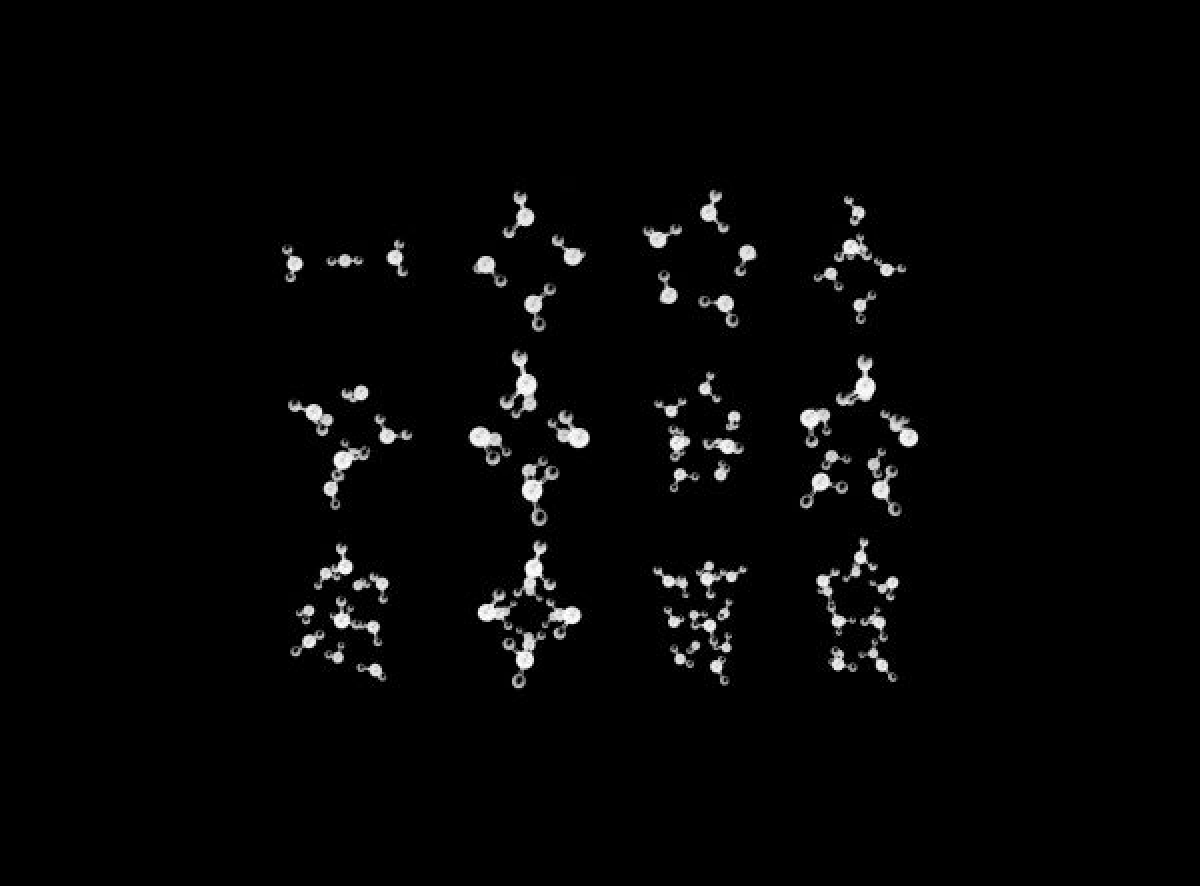,

November 25, 2021T.E. O'Brien et al. (Google, Boehringer Ingelheim, Macquarie, PNNL)

Quantum computing has been progressively applied in the field of quantum chemistry, especially applying modern computational approaches to navigate molecular potential energy surfaces. One such problem is calculation of forces, i.e. calculating the derivative of energies with respect to nuclear positions. These calculations are usually done by Molecular Dynamics (MD) simulations, and are used in multiple applications such as the description of heterogeneous processes on surfaces including catalysis, observation of phase transitions (e.g. nucleation processes for water), and in pharmaceutical research (observation of the interaction of drugs with their targets in the human body).

So far, quantum algorithms like force estimation via the Hellman-Feynman theorem and quantum gradient estimation have been implemented experimentally. However, these algorithms suffer from limited accuracy in a fault-tolerant or NISQ setting. There has been theoretical work that addresses the theoretical chemistry behind force estimation on a quantum device, presenting a detailed derivation in a Lagrangian formalism focusing on an ab initio exciton model, and stressed the importance of including full response. Also, the mathematical formulation of force estimation in NISQ on a significantly stronger footing has been provided, which combined this with gradient estimation for the optimization of variational quantum eigensolvers. The progress also includes small-scale experimental implementations.

In this work, the authors propose new quantum algorithms and resource estimates for computing molecular energy derivatives. The objective is to optimize and cost (value) methods for estimating forces and other first-order energy gradients for NISQ and fault-tolerant quantum computers. Firstly, the work estimates energy derivatives via the Hellman-Feynman theorem presenting a simple, calculable derivation of the force operator in second quantization for an atomic-centered basis orbital set, based on the orbital connection theory of Helgaker and Almlöf. Following that, the work derives the exact form of the force operator in a plane wave basis in first- and second-quantization. In order to estimate the error tolerance on a force vector, radial distribution function calculations were carried out for a system of 216 water molecules. The results demonstrate that for relevant molecular dynamics and geometry optimization, an RMS of the error in one force component should be below a certain threshold (0.6 mHa/Å).

Secondly, in order to estimate force vectors in molecular systems, the authors employ the state tomography method and optimization assuming that the preparation of an initial state satisfies the Hellman-Feynman theorem. The work shows calculations of the relevant bound on the cost of the number of measurements to estimate a constant 2-norm error, both analytically and numerically for hydrogen chains along with the estimation of the asymptotic costing for both. Since block-encoding is a required step in FTQC, the work proposed two methods - finite-difference algorithms for computing gradients as well as another method based on estimating expectation values of force operator to block-encode Hamiltonians and force operators in second quantization for factorized methods, and in first quantization for plane waves. The finite-difference algorithm is shown to provide asymptotically better scaling where the expected cost of state preparation is low, whereas in the case of high state preparation cost, the gradient operator approach provides better scaling. Moreover, the cost of simulating forces is similar to the cost of simulating Hamiltonians using sparse simulation methods, but for factorized methods, the cost is clearly asymptotically lower.

Practically speaking, the MD simulations require on the order of 106 − 109 unique force calculations estimating multiple hours of computing time for single-point energy calculations on fault-tolerant devices. An important result of this work is to show that such numbers are impractically large and prohibit immediate quantum advantage to be obtained with current generation hardware and methods.

Further research in force optimization can be done in improving the bounds on the variance obtained for NISQ systems. Moreover, it would be highly advantageous to perform a fully-quantum simulation as opposed to a semi-classical one, to avoid the costly gradient computation step. One approach could be simulating the differential equation governing the dynamics on the quantum computer itself, while retaining a classical description of the nuclear motion within the Born-Oppenheimer approximation, or by a fully-quantum simulation that treats the nuclei and electrons on a similar footing such as a first quantized simulation. Alternatively, taking a small number of measurements from a quantum device and fitting a semi-empirical model to the results can also be considered. This could be done using a semi-classical molecular mechanical model. Finally, one could consider reusing points from finite difference calculations, or sparsely sampling gradients to reduce these constant factor overheads. To conclude, it’ll be interesting to monitor progress in this field to properly predict when and where quantum computers could provide benefits in the computation of molecular forces and in-general energy gradients.